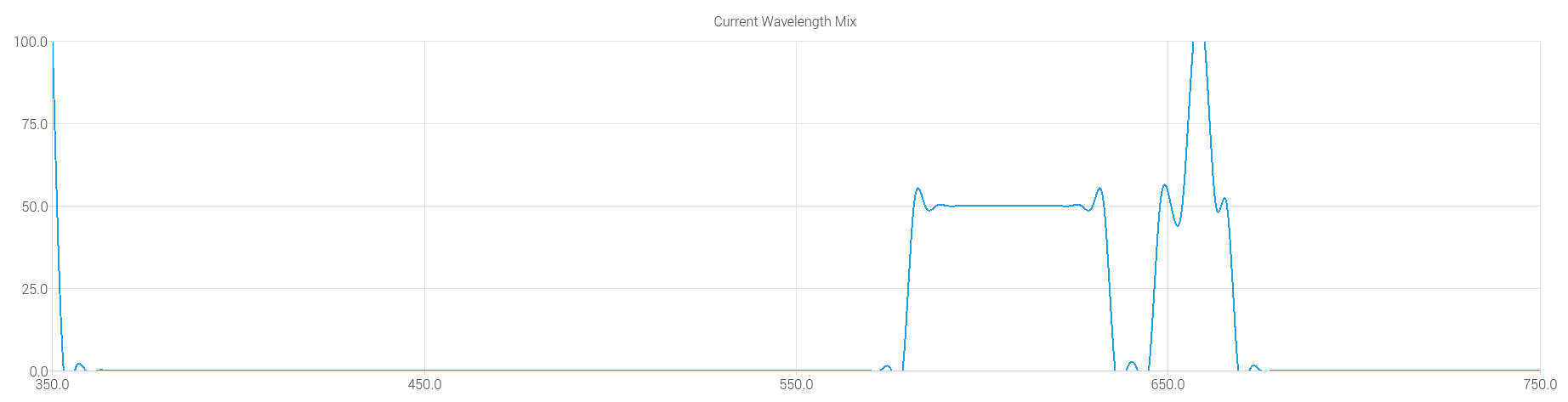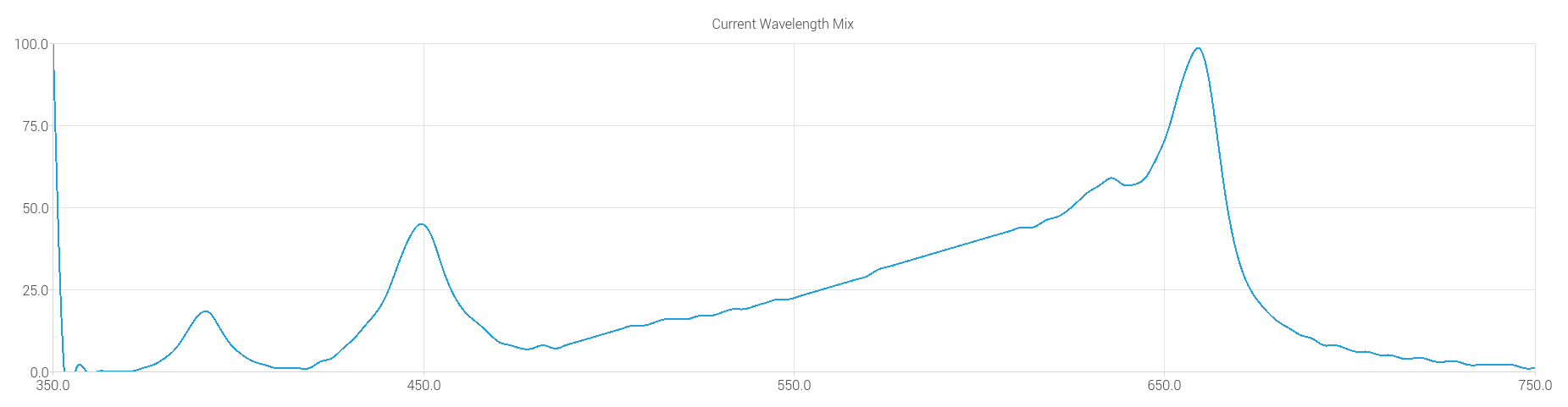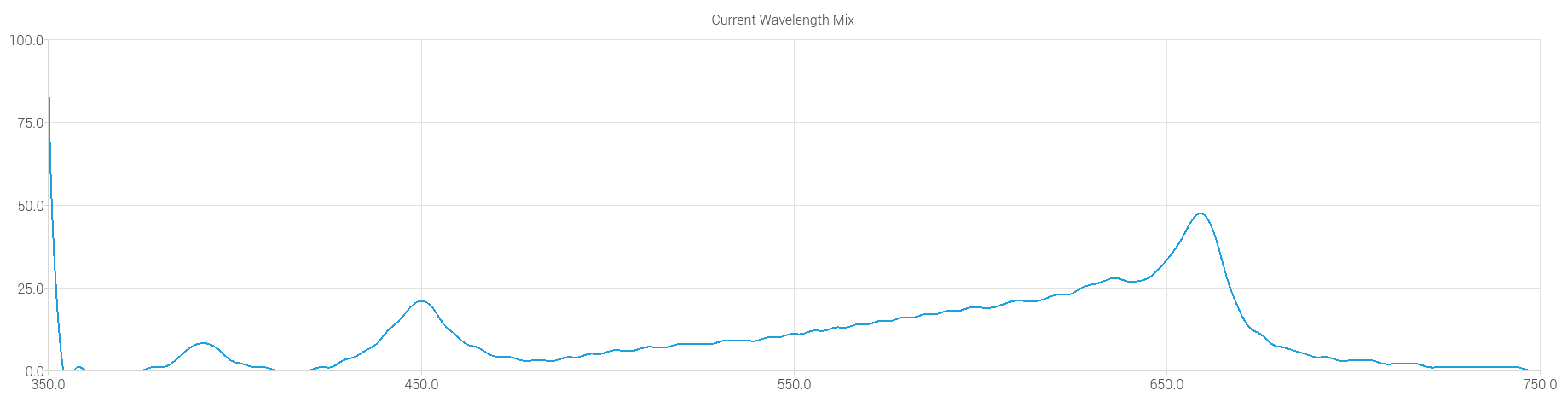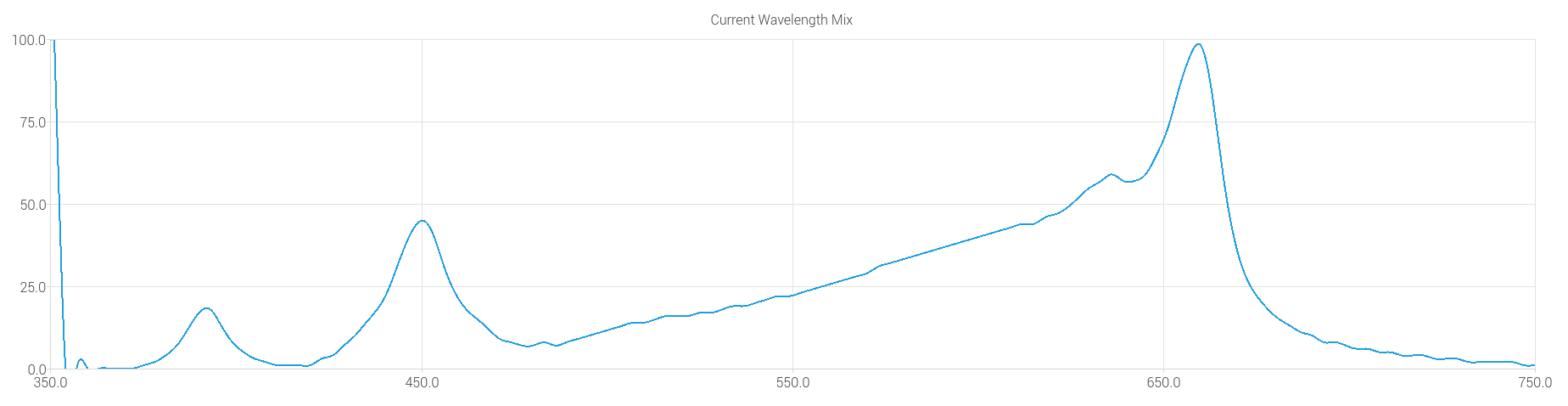# QChart Axes auto-resizing without telling me

• Hello
I'm working on a Qchart with a dynamic spline graph. The graph is displaying a range of wavelengths, and by moving sliders up and down, you can see the amplitude of light at each wavelength. The maximum amplitude contribution of each slider is preset and hard-coded, the slider just determines what percent each one is at.

The problem I'm having is that the graph zooms itself way in - this took me a lot of time to realize it was the graph and not my math! So you can see in the first two pictures below, the graph is very blocky and terrible, which is just a super zoomed-in version of my graph, so you can see the interpolation happening on a microscale. For the next two pictures, I appended a point before the rest of the data set to (350, 100) to force the graph to be scaled properly. This shows the graph correctly, where the lines are able to be under the maximum without the graph zooming in.

Here's how I'm creating the graph initially. I assumed (apparently incorrectly) that setting the range of the axes would mean they were static, but it appears those numbers are useless and no better than arbitrary labels? I'm hoping there's a better way to create a static axis that doesn't auto-update based on the data.

``````//Spline Graph
initialSplineSeries = new QSplineSeries();
initialSplineSeries->setName("initial spline series");
for(int i = 0; i < 134; ++i)
{
initialSplineSeries->append(wavelength_arr[i], 0);
}
splineChart = new QChart();
splineChart->legend()->hide();
splineChart->setTitle("Current Wavelength Mix");
splineChart->createDefaultAxes();
splineChart->axes(Qt::Vertical).first()->setRange(0, 100);
splineChart->axes(Qt::Horizontal).first()->setRange(351, 750);
splineChartView = new QChartView(splineChart);
splineChartView->setSizePolicy(QSizePolicy::Expanding, QSizePolicy::Expanding);
``````

And then inside a function called by the sliders is where I generate the new lineseries: you can also see the commented line where I placed the scaling point at the beginning to force the graph.

``````void DimmerWidget::update_graph()
{
splineChart->removeAllSeries();
newSplineSeries = new QSplineSeries();
newSplineSeries->setName("fresh series");
//newSplineSeries->append(350, 100);
int white_cont;
int red_cont;
int blue_cont;
int total_cont;
for(int i = 0; i < 134; ++i)
{
white_cont = (white_arr[i] * dimm_currVal_int) / 1000000;
red_cont = (red_arr[i] * dimm_currVal_int) / 1000000;
blue_cont = (blue_arr[i] * dimm_currVal_int) / 1000000;
total_cont = white_cont + red_cont + blue_cont;
newSplineSeries->append(wavelength_arr[i], total_cont);
}
}
``````

Auto-scaled graph with very small values, very zoomed-in.Same auto scaled graph, but less zoomed in.Graph with a point in the beginning to force the scaling, note how the peak in the middle is not touching the top.Same graph at the maximum.• I'm back, and I solved it!

I'll leave the answer here for anyone else who runs into this. So the problem was the axis creation method I was using, even though that's in an example. Probably because I was using dynamic data that was going to change it's range, the example didn't exactly cover that. I'm now creating axes the proper way, and the key here is that the axes are then attached to the data, both when I initialize the graph, and each time I change the lineseries in my update_graph() function.

I finally found a guy with a similar problem, and was able to base my solution off the answer given. Thanks guy from 2018 on Stack Overflow!

My modified code is below:

``````//Spline Graph
initialSplineSeries = new QSplineSeries();
initialSplineSeries->setName("initial spline series");
for(int i = 0; i < 134; ++i)
{
initialSplineSeries->append(wavelength_arr[i], 0);
}
splineChart = new QChart();
splineChart->legend()->hide();
splineChart->setTitle("Current Wavelength Mix");
axisX = new QValueAxis;
axisY = new QValueAxis;
axisX->setRange(351, 750);
axisY->setRange(0, 100);
splineChartView = new QChartView(splineChart);
splineChartView->setSizePolicy(QSizePolicy::Expanding, QSizePolicy::Expanding);
splineChart->setAxisX(axisX);
splineChart->setAxisY(axisY);
initialSplineSeries->attachAxis(axisX);
initialSplineSeries->attachAxis(axisY);
``````
``````void DimmerWidget::update_graph()
{
splineChart->removeAllSeries();
newSplineSeries = new QSplineSeries();
newSplineSeries->setName("fresh series");
int white_cont;
int red_cont;
int blue_cont;
int total_cont;
for(int i = 0; i < 134; ++i)
{
white_cont = (white_arr[i] * dimm_currVal_int) / 1000000;
red_cont = (red_arr[i] * dimm_currVal_int) / 1000000;
blue_cont = (blue_arr[i] * dimm_currVal_int) / 1000000;
total_cont = white_cont + red_cont + blue_cont;
newSplineSeries->append(wavelength_arr[i], total_cont);
}